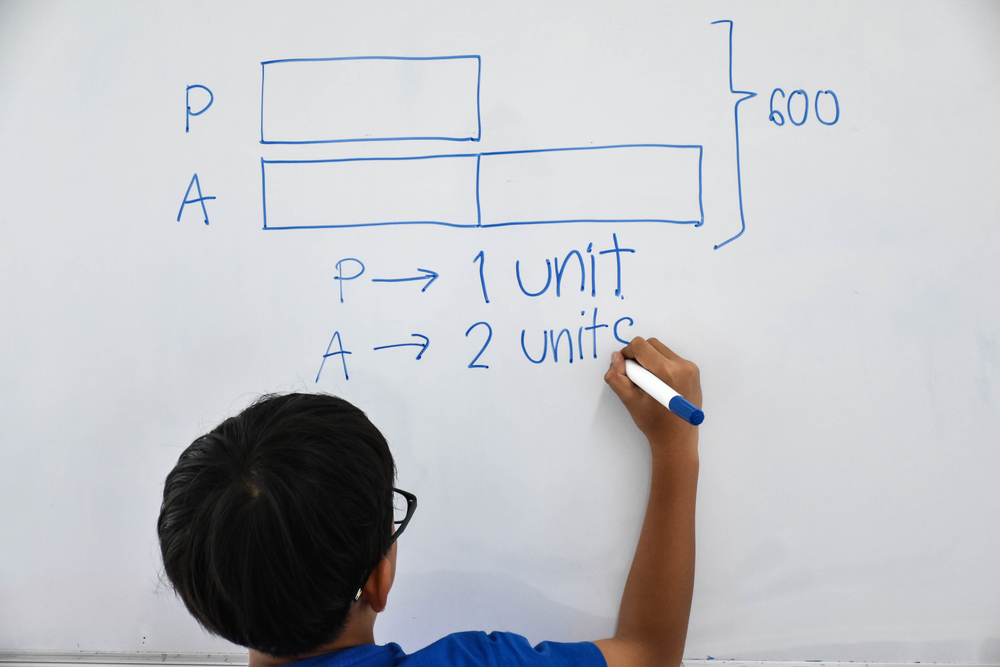All Posts

# Ways to Use Visual Learning in Elementary Math

June 4, 2018

Once considered an unintelligent way to solve math problems, researchers now believe that using fingers to count helps solidify representations of numbers in our minds, making kids and adults alike more successful in math. Like counting fingers, there are many other visual techniques, or models, to use to help kids understand numbers and basic operations.

Since many kids are visual learners, it’s important that they are exposed to different visual math models to increase their understanding of math, setting them up for success as they continue their studies. Let’s start with the basics of visual math before moving on to explore easy study tips for visual learners.## What is Visual Math?

Visual math models are tools that teachers and parents can use to help kids understand math concepts. Visual math models can be as simple as using pizza slices to represent fractions, or they can be diagrams or graphs provided on paper meant to offer a visual representation of a math concept.

Because math can be abstract, forcing kids to count and imagine large numbers, models are used to help kids learn to visualize concepts, bringing clarity to a complex math operation, like multiplication, or place value using decimals.

## Why is Visual Math Important?

When kids read a story, they learn to picture the events in their minds, which in turn helps kids boost comprehension and understanding of the setting, characters, plot, and more. Likewise in math, kids can understand a concept better if they can form a mental picture of the idea being studied.

#### Special Offer: Get 60% off the yearly subscription on Kids Academy: Talented & Gifted Program! You’ll find math games and videos that feature the below math models, and so much more!

In fact, recent studies have shown that the brain uses multiple regions while kids complete math, including visual and sensory motor pathways. Therefore, math for visual learners should include concrete representations that kids can see to aid in their understanding of the underlying math concept.

## Visual Math Models to Use at Home or in the Classroom

In the meantime, look for the following visual math models to deepen your child’s understanding of early elementary math concepts:

### Base Blocks

These are the ultimate in visual math tools, as they can serve as a powerful model, as well as a worthwhile manipulative, to help your child understand large numbers and place value up through the thousands. When kids start out learning place value, they need a way to easily understand the concept of ones, tens, and hundreds.

Base blocks makes this easy by showing kids what groupings of large numbers look like, offering an a simple way to see and understand place value.

### Number Lines

Linear representations help kids understand each number’s place on an infinite continuum. Moreover, they help kids learn to add and subtract easily. Number lines also develop mental math strategies, and after kids master using them, they are able to visualize them in their minds for quick problem solving.

### Arrays

Arrays are useful tools that are used in conjunction with teaching kids beginning multiplication. While you might remember memorizing multiplication tables, kids can also use arrays to understand the process behind multiplication. Displayed as a grid of dots or small images, kids can count the dots horizontally and vertically to find the multiplication sentence, before counting all of them to understand the answer.

### The 100 Square

Hundred squares are used to help kids find different types of patterns. Kids can investigate even and odd numbers, add, subtract, multiply, or divide, or even use blank charts to explore and explain decimals. With so many uses, the 100 square is a truly versatile visual model that can benefit learners no matter their current math level!

Using visual math models is a powerful way to reinforce your child’s math learning, while providing a solid way to develop important mental math strategies. Look for interactive games and printable worksheets that feature the above visual math models, and prime your child for continued math success!

Mobile version
SHARE